Courses

# Stability Analysis Of Linear Control Systems

## 10 Questions MCQ Test Mock Tests Electronics & Communication Engineering GATE 2020 | Stability Analysis Of Linear Control Systems

Description
This mock test of Stability Analysis Of Linear Control Systems for GATE helps you for every GATE entrance exam. This contains 10 Multiple Choice Questions for GATE Stability Analysis Of Linear Control Systems (mcq) to study with solutions a complete question bank. The solved questions answers in this Stability Analysis Of Linear Control Systems quiz give you a good mix of easy questions and tough questions. GATE students definitely take this Stability Analysis Of Linear Control Systems exercise for a better result in the exam. You can find other Stability Analysis Of Linear Control Systems extra questions, long questions & short questions for GATE on EduRev as well by searching above.
QUESTION: 1

Solution:
QUESTION: 2

Solution:
QUESTION: 3

### The number of sign changes in the Routh’s array indicates the number of roots lying in the

Solution:

Number of sign changes in the first column of Routh’s array indicates the number of closed loop poles or roots of characteristic equation lying in the right half of s-plane.

QUESTION: 4

Assertion (A): A system is said to be stable if the impulse response approaches zero for sufficiently large time.
Reason (R): If the impulse response approaches infinity for sufficiently large time, the system is said to be unstable

Solution:

Both assertion and reason are individually true. The correct reason for assertion is BIBO stability criteria,

QUESTION: 5

The open loop transfer function of a unity feedback system is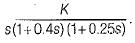What is the range of value of K so that the closed loop system is absolutely stable?

Solution:

The characteristic equation is,
1 + G(s)H(s) = 0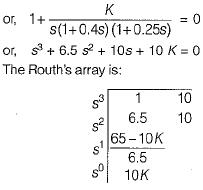For absolute stability, we have: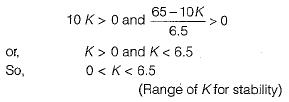QUESTION: 6

Assertion (A): If the system is stable we can determine the relative stability by the settling time of the system.
Reason (R): If the settling time is less than that of the other system then the system is said to be relatively more stable.

Solution:

The settling time is inversely proportional to the real part of the dominant roots. If the settling time is less compared to other system, then the system will be relatively more stable

QUESTION: 7

If the unit step response of a system is given by c(t) = -1.5 + et + 0.5e-2f, then the system will be

Solution:

Given, step response: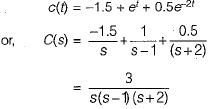∴  Transfer function is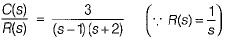Since one of the closed loop pole (s = 1) lies in RH s-plane, therefore given system is unstable.

QUESTION: 8

The characteristic equation of a servo system is given by
a0s4 + a1s3 + a2s2 + a3s + a4 = 0
Now, consider the following conditions required to be satisfied by the coefficient of the above characteristic equation for the system to be stable:
1. a1 > 0 and a4 > 0
2. a1 < 0 and a4 > 0
3. a1a2< a0a3
4. a1a2 > a0a
5.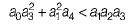6.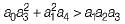Which of the above conditions holds true for the given system to be stable?

Solution:

The Rouths array is formed as follows: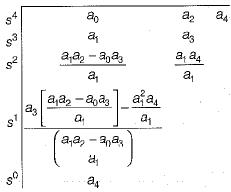For the system to be stable, there should not be any sign change in the first column of Routh’s array.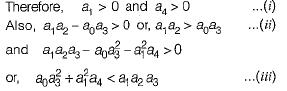Hence, above conditions must hold for the given system to be stable.

QUESTION: 9

The open loop transfer function of a unity feedback control system is given by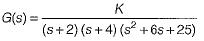​The oscillating frequency of the given system is

Solution:

The characteristic equation of given system is: 1 + G(s) = 0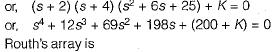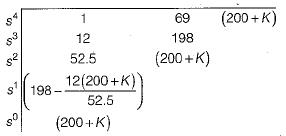Given system will be stable when,
200 + K > 0 or K > -200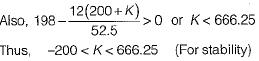System will oscillate when K = Kmar = 666.25
Auxiliary equation is : 52.5 s2 + (200 + K) = 0
or, 52.5 s2 = - 866.25 or, s2 = -16.5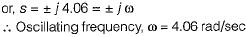QUESTION: 10

The characteristic equation of a system is given by s3 + s + 2 - 0.

What are the number of roots in the right half s-plane and on the jω-axis respectively?

Solution:

Routh’s array is formed as shown below,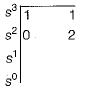Replacing ε in place of 0 in 1st column, we have: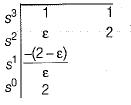To check for sign change: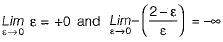Thus, there are two sign changes in 1st column of Routh’s array (+0 to -∞ and -∞ to + 2).
Hence, number of roots in RH s-plane = 2.
Number of roots in LH s-plane = 1.
Number of roots on the jω-axis = 0.